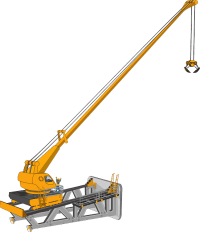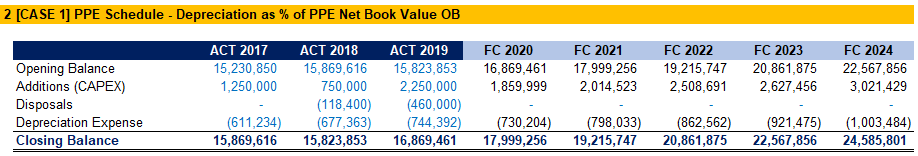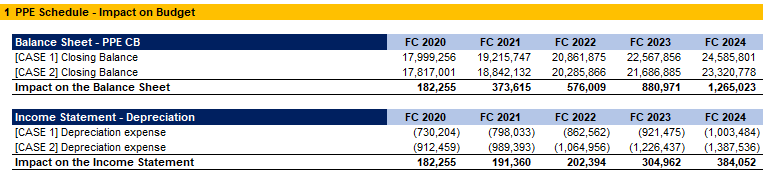## CAPEX, Depreciation and Amortization in Financial Modeling

If you are already familiar with the outlined concepts, maybe you would be more interested in taking a look at the Excel model, which you can download below the article.

## Introduction

Long-term (non-current) assets of the company have a long useful life (more than one year). When acquiring capital assets, we aim to use them within the business and not hold them for re-sale. These primarily consist of land, buildings, fixtures and fittings, equipment and machinery, needed to operate the business.When performing a valuation or preparing a financial model, one set of essential assumptions we need to make, have to do with the long-term assets of the company, namely Property, Plant and Equipment (PPE), and Intangible assets. For this purpose, we have to forecast capital expenditures for acquiring new assets (Capex), as well as depreciation and amortization. We use such assumptions in both the Discounted Cash Flow (DCF) model and the Capitalization of Cash Flow model.

## Capital Expenditures (Capex)

Capex is the total expenditure on the purchase of assets by the business in a given period. This includes both assets acquired and built by the company.

Capital assets provide value to the business over a period, longer than one reporting period.

`CAPEX = Net Increase in PPE + Depreciation Expense`

Where

`Net Increase in PPE = PPE Closing Balance - PPE Opening Balance`

Capital expenditures are reflected in the Property, Plant & Equipment in Non-current assets on the Balance sheet. We also include the Capex, which we pay during the period in the Cash Flow Statement.

### Forecasting Capex

When we budget the capital expenditures, we need to be in line with the other financial projections for the company. If we plan to increase sales revenue and increase the number of employees to achieve expansion, we need to plan Capex to support the growth. This may include acquiring new offices, working stations, computers, and others, or even new production capacities, to achieve the sales target.Capex estimations are never 100% sure. We are making an educated guess at their value, based on available information and knowledge, to arrive at a realistic estimate.

Sales revenue is a typical driver for Capex in financial modeling. We reference historical capital expenditures to project future spending on capital assets. Another method is to calculate an average and plan a fixed Capex amount per period. In some cases, we might have a detailed program for capital investments, which we can use in our forecast.

Keep in mind that Capex always comes before depreciation and amortization in our models, as the company cannot depreciate assets before acquiring them.

## Depreciation and Amortization

Long-term assets are depreciated or amortized over time, and we present the remaining net book value (NBV) in the Balance sheet.

Depreciation occurs when the business uses up fixed assets. Physical assets used for more than a year degrade over time and lose value. The same happens with Intangible assets, where amortization is charged, to show how the asset is transferring its value into the business operations.

Different assets lose value at different rates, based on their intrinsic useful lives. Fixed asset registers help outline these differences and calculate appropriate depreciation and amortization expenses. These schedules usually include information on the type of asset, depreciation method used, useful life, book value (cost of acquisition), accumulated depreciation, net book value (book value less accumulated depreciation), and others.

### Forecasting Depreciation and Amortization

To estimate the charges for depreciation and amortization, we start by understanding how assets reduce their value over time. We look into historical data, analyze the useful lives, applied depreciation methods, and the existence of long-lived assets like buildings.

Based on our understanding of the industry and the business, we can forecast depreciation based on various assumptions. We can calculate the charge as a % of Capex, the Net Book Value of the assets, or even sales revenue, based on the historical trends we identify. We can also roll a fixed amount, especially for companies with low to no capital expenditures, or apply a reasonable growth rate to the historical depreciation and amortization expenses.

If we notice that the charges have remained stable over the past periods, this may indicate the company uses a straight-line method to calculate depreciation and amortization. We can then calculate the expense as a percentage of the NBV of the assets, or roll a fixed amount.

When calculating our forecasted depreciation schedule, we need to ensure that the accumulated depreciation does not exceed the book value of the asset, as this will result in a negative net asset value, which is not possible in reality. To achieve this, we calculate accumulated depreciation as the smaller of:

`Accumulated Depreciation Opening Balance + Current Year Depreciation Charge`

or

`Initial Book Value of the Asset`

## Example

To emphasize the importance of setting proper assumptions, let us look at an example forecast of a Property, Plant and Equipment schedule.

We have historical data for the years 2017 to 2019. The task at hand is to forecast the PPE balance, CAPEX, and Depreciation expense for the next five years, to support management’s decision-making process. We will start with our assumptions table. We have the Sales revenue and Depreciation expense for the past three years, as well as the forecasted Sales for the next five years. We do not have a detailed CAPEX plan, so we decide to forecast CAPEX as a percentage of Sales. Calculating a moving average of three periods, we arrive at the rates we will apply in our forecast. As we do not have a detailed Depreciation schedule of all assets, we estimate the expense as a percentage of the opening balance of the net value of PPE.Now that we have our assumptions figured out, we can apply the rates in our PPE Schedule.This is enough for a five-year plan, as we understand it’s not possible to estimate the actual values, and it is OK if they deviate from our forecast.

However, we notice that if we calculate depreciation as a percentage of sales for the three years of available data, we get a more consistent rate.Following the same logic, we prepare a second case for our PPE Schedule, applying the assumption that depreciation is calculated based on sales, and not opening balance of PPE.The resulting PPE schedule is different from the first one we prepared. Let us examine the deviation and what impact it would have on our forecasted financial statements.

The changes apply to Depreciation expense and PPE closing balance, so let us look at how these two impact our forecast. We include the PPE closing balance in the Balance sheet. By comparing the two cases, we see that we will have a consistently lower balance if we apply the second set of assumptions. This will change our balance sheet lines for Non-current assets and Total assets and will have an impact on various financial analysis ratios we might calculate.

Looking at the Depreciation expense, in the second case, we are estimating a consistently higher charge, which will impact our bottom line, meaning we will be forecasting lower profits for all periods under our second set of assumptions. This deviation will also have an impact on several performance metrics.We need to look further into the Property, Plant and Equipment of the company to support our choice of assumptions. It is important to remember that it is easy to perform the calculation part of an estimation. Arriving at the proper conclusion for the assumptions we select is the hard part; it is a form of art.

## Conclusion

Capital expenditures and Depreciation & Amortization are fundamental forecast assumptions in the financial modeling and valuation processes. We need to estimate those metrics to forecast the fixed assets in the Balance Sheet, the depreciation and amortization expense in the Income Statement, and the Capex in the Cash Flow Statement. We need to be aware that we can never achieve a 100% accuracy, and it’s easy to spiral down into calculations that are too detailed for the purpose at hand. To mitigate this risk, we have to obtain an adequate understanding of the industry and the company.

## And Get a FREE Benchmark Analysis Template## FCCA, FMVA

Hi! I am a finance professional with 10+ years of experience in audit, controlling, reporting, financial analysis and modeling. I am excited to delve deep into specifics of various industries, where I can identify the best solutions for clients I work with.

In my spare time, I am into skiing, hiking and running. I am also active on Instagram and YouTube, where I try different ways to express my creative side.

### 2 Responses

1. FELIPE DE AMORIM CARVALHO says:

I Understand the difference between the 2 cases and results, but it was not clear to me which one is the right approach ? Or when do I use one or another?

1. Dobromir Dikov says:

Hi Felipe,